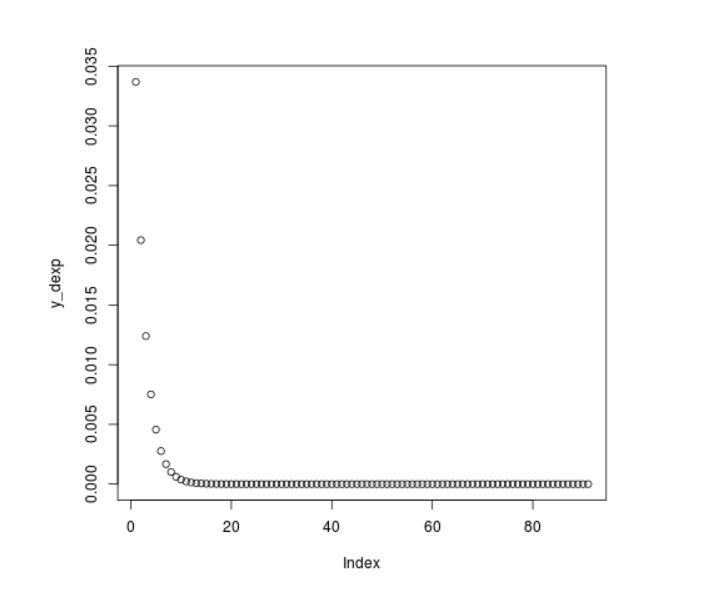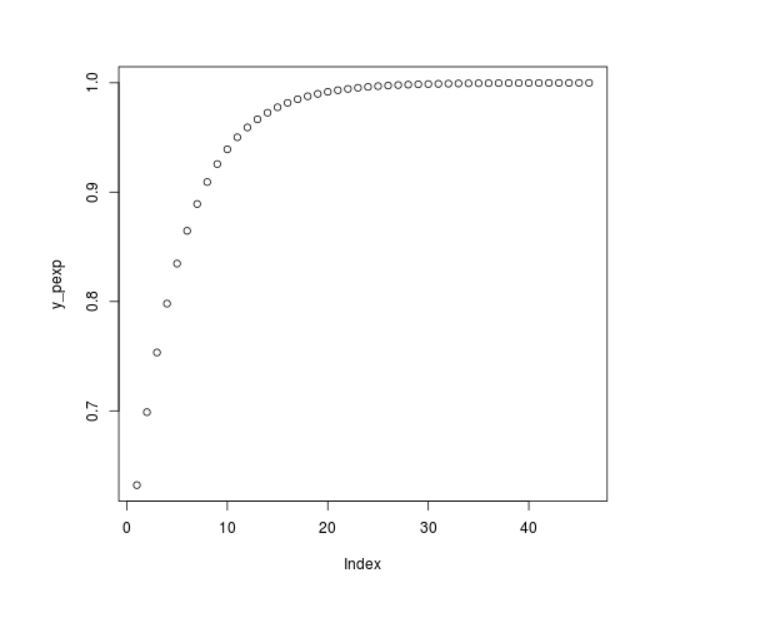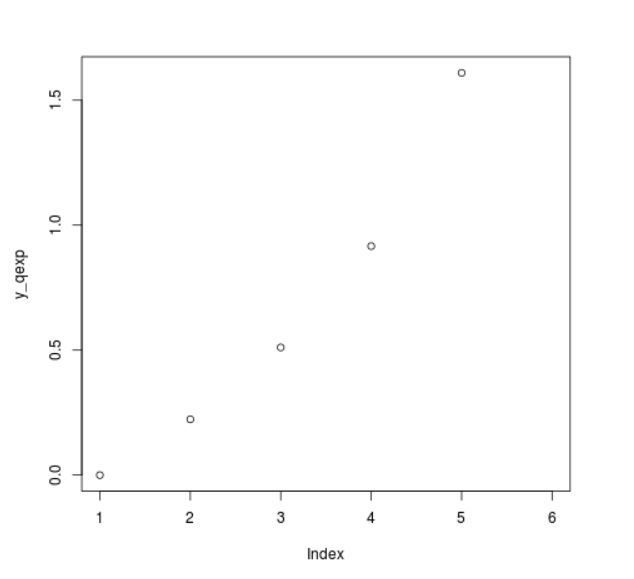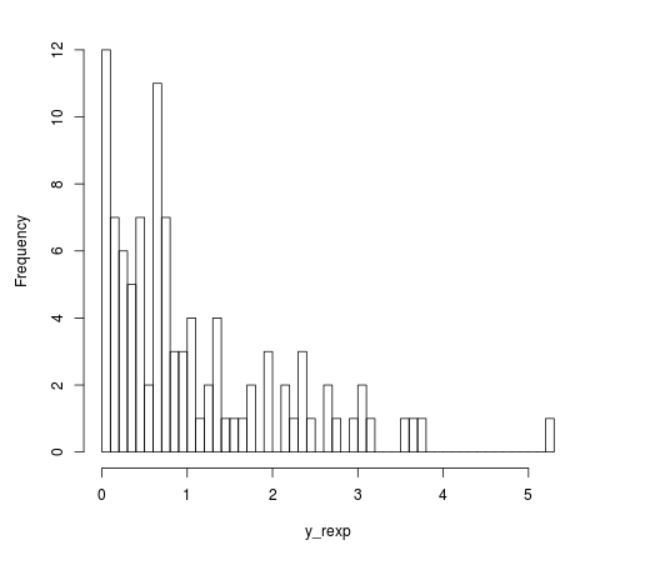# Exponential Distribution in R Programming – dexp(), pexp(), qexp(), and rexp() Functions

The exponential distribution in R Language is the probability distribution of the time between events in a Poisson point process, i.e., a process in which events occur continuously and independently at a constant average rate. It is a particular case of the gamma distribution.

In R, there are 4 built-in functions to generate exponential distribution:

• dexp()
`dexp(x_dexp, rate) `
• pexp()
`pexp(x_pexp, rate )`
• qexp()
`qexp(x_qexp, rate)`
• rexp()
`rexp(N, rate )`

where,

x: represents x-values for exp function .
rate: represents the shapex.
N: Specify sample size

### Functions To Generate Exponential Distribution

#### dexp() Function

`dexp()` function returns the corresponding values of the exponential density for an input vector of quantiles.

Syntax:

`dexp(x_dexp, rate)`

Example:

 `# R program to illustrate ` `# exponential distribution ` `# Specify x-values ` `x_dexp <``-` `seq(``1``, ``10``, by ``=` `0.1``)  ` `      `  `# Apply dexp() function                ` `y_dexp <``-` `dexp(x_dexp, rate ``=` `5``)     ` `                `  `# Plot dexp values  ` `plot(y_dexp) `

Output:#### pexp() Function

`pexp()` function returns the corresponding values of the exponential cumulative distribution function for an input vector of quantiles.

Syntax:

`pexp(x_pexp, rate )`

Example:

 `# R program to illustrate ` `# exponential distribution ` ` `  `# Specify x-values ` `x_pexp <``-` `seq(``1``, ``10``, by ``=` `0.2``)                                      ` ` `  `# Apply pexp() function ` `y_pexp <``-` `pexp(x_pexp, rate ``=` `1``)  ` ` `  `# Plot values                   ` `plot(y_pexp)                                                     `

Output :#### qexp() Function

`qexp()` function gives the possibility, we can use the qexp function to return the corresponding values of the quantile function.

Syntax:

`qexp(x_qexp, rate)`

Example:

 `# R program to illustrate ` `# exponential distribution ` ` `  `# Specify x-values  ` `x_qexp <``-` `seq(``0``, ``1``, by ``=` `0.2``)                      ` `  `  `# Apply qexp() function ` `y_qexp <``-` `qexp(x_qexp, rate ``=` `1``) ` `  `  `# Plot values                    ` `plot(y_qexp)                                        `

Output:#### rexp() Function

`rexp()` function is used to simulate a set of random numbers drawn from the exponential distribution.

Syntax:

`rexp(N, rate )`

Example:

 `# R program to illustrate ` `# exponential distribution ` ` `  `# Set seed for reproducibility ` `set``.seed(``500``)  ` ` `  `# Specify size          ` `N <``-` `100` ` `  `# Draw exp distributed values ` `y_rexp <``-` `rexp(N, rate ``=` `1``) ` `  `  `# Plot exp density   ` `hist(y_rexp, breaks ``=` `50``, main ``=` `"") `

Output :My Personal Notes arrow_drop_upCheck out this Author's contributed articles.

If you like GeeksforGeeks and would like to contribute, you can also write an article using contribute.geeksforgeeks.org or mail your article to contribute@geeksforgeeks.org. See your article appearing on the GeeksforGeeks main page and help other Geeks.

Please Improve this article if you find anything incorrect by clicking on the "Improve Article" button below.

Article Tags :

Be the First to upvote.

Please write to us at contribute@geeksforgeeks.org to report any issue with the above content.